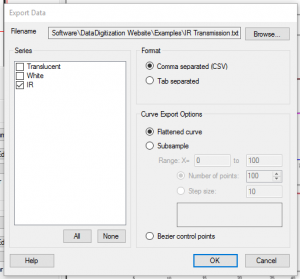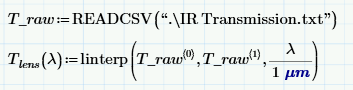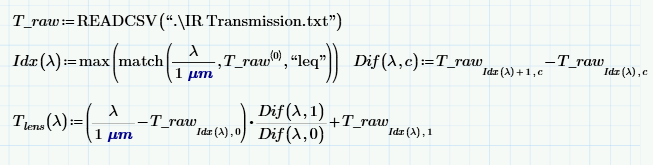Dagra was used in this example to trace the transmittance of a Fresnel lens. The goal of the project was to estimate how much energy from the skin of a person would be focused onto an infrared sensor. The lens had a lot of strong absorption bands in the wavelengths we were interested in so tracing the transmission from the manufacturer’s datasheet helped provide a more accurate model.

Once the lens transmission data was traced using Dagra, the free version of MathCAD was used to calculate the power falling on the sensor.

The files — including the Dagra project, exported data and MathCAD document — for this example can be downloaded using the link below.

## Black Body Equation

As we are interested in energy in infrared wavelengths, skin can be treated as a black-body source (to a first approximation).

The spectral radiance of a black-body, the energy density emitted at each wavelength, can be modeled by Plank’s law. Plank’s law describes the spectral radiance, $latex I(\lambda, T)$ emitted by a black-body at some temperature $latex T$ and wavelength $latex \lambda$ as:
$latex I(\lambda, T) =\frac{2 hc^2}{\lambda^5}\frac{1}{ e^{\frac{hc}{\lambda k_\mathrm B T}} – 1}$

Here $latex k_B = 1.381 \cdot 10^{-23} \frac{J}{K}$ is Boltzmann’s constant, $latex h= 6.626 \cdot 10^{-34} J\cdot s$ is Plank’s constant and $latex c = 2.9979 \cdot 10^8 \frac{m}{s}$ is the speed of light in a vacuum.

## Lens and Detector Properties

The signal measured by the detector will depend on the area of the detector, the wavelengths it is sensitive to, the numerical aperture of the lens and the temperature of the skin.

In the example problem we have:

• $latex Area = 5 mm^2$: Area of the detector
• $latex NA = 0.2$: Numerical aperture of the lens in front of the detector
• $latex \lambda_{min} = 7 \mu m$: Minimum wavelength the detector is sensitive to
• $latex \lambda_{max} = 13 \mu m$: Maximumwavelength the detector is sensitive to
• $latex T_{source} = 273.15 K + 38 K$: The temperature of the skin

The transmission of the lens material traced using Dagra. To use the traced data in MathCAD, first export it as comma separated values. Select File → Export data… in Dagra. Select the series to export, a filename and comma separated (CSV) as the format.Use the comma separated (CSV) format to export data that will be used in MathCAD

Use MathCAD’s READCSV function to load the exported data. The data as loaded as a matrix. The first column of the matrix contains the x-values traced by Dagra and the second column contains the y-values.

Its usually more convenient to work with a function that interpolates the digitized data and returns the value for a specific x-value. If you have the professional version of MathCAD you can use linterp, a built-in function for linear interpolation. The function definition for calculating the transmission of the lens ($latex T_{lens}$) for some wavelength, $latex \lambda$, is:If you have the free version of MathCAD, it is still possible to create an equation to return the lens transmission but the interpolation has to be done manually:## Calculate the Power on the Detector

The power falling on the detector is an integral over all the wavelengths it is sensitive to. We integrate the power from the source, filtered by the lens. The numerical aperture and detector area are constants that scale the result:

$latex Power = NA^2 \cdot Area \cdot \int_{\lambda_{min}}^{\lambda_{max}} I(\lambda, T_{source}) \cdot T_{lens}(\lambda) d\lambda = 7.2 \mu W$

Not readable? Change text.